# Examples#

Examples demonstrating connectivity analysis in sensor and source space.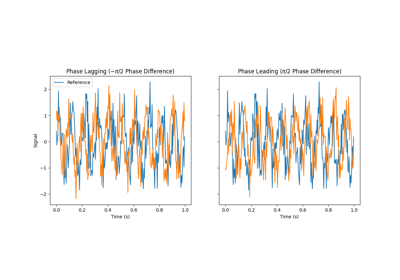Comparing PLI, wPLI, and dPLI

Comparing PLI, wPLI, and dPLI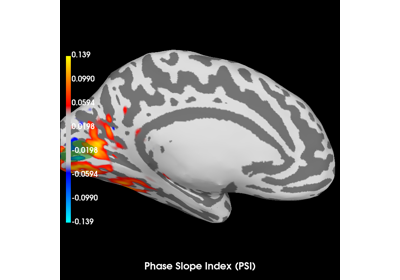Compute Phase Slope Index (PSI) in source space for a visual stimulus

Compute Phase Slope Index (PSI) in source space for a visual stimulus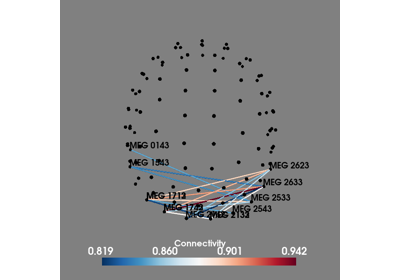Compute all-to-all connectivity in sensor space

Compute all-to-all connectivity in sensor space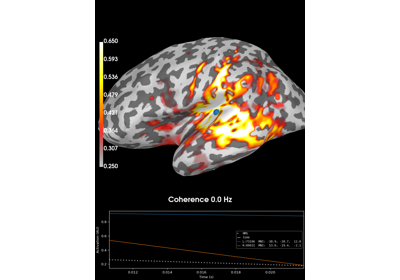Compute coherence in source space using a MNE inverse solution

Compute coherence in source space using a MNE inverse solution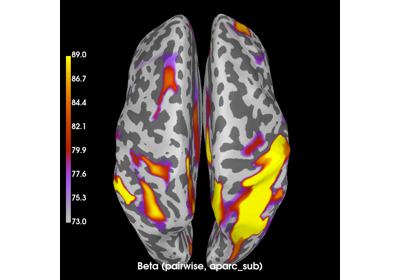Compute envelope correlations in source space

Compute envelope correlations in source space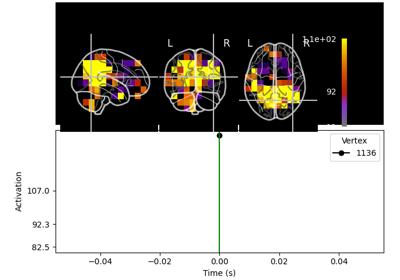Compute envelope correlations in volume source space

Compute envelope correlations in volume source space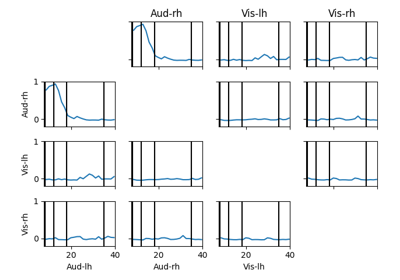Compute full spectrum source space connectivity between labels

Compute full spectrum source space connectivity between labels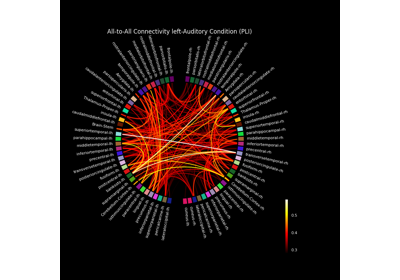Compute mixed source space connectivity and visualize it using a circular graph

Compute mixed source space connectivity and visualize it using a circular graph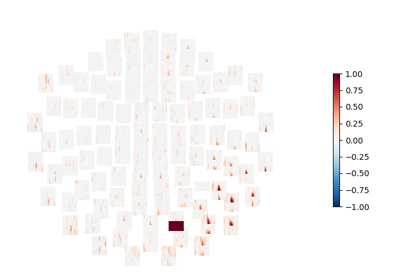Compute seed-based time-frequency connectivity in sensor space

Compute seed-based time-frequency connectivity in sensor space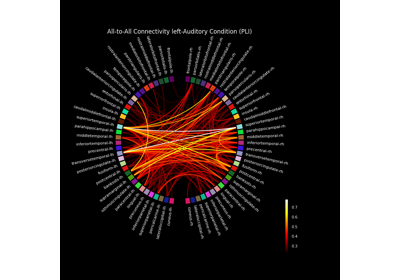Compute source space connectivity and visualize it using a circular graph

Compute source space connectivity and visualize it using a circular graphUsing the connectivity classes

Using the connectivity classes

# Dynamic Connectivity Examples#

Examples demonstrating connectivity analysis with dynamics. For example, this can be a vector auto-regressive model (also known as a linear dynamical system). These classes of models are generative and model the dynamics and evolution of the data.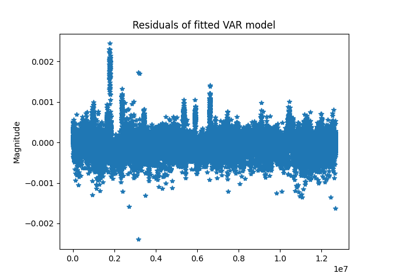Compute vector autoregressive model (linear system)

Compute vector autoregressive model (linear system)

Gallery generated by Sphinx-Gallery